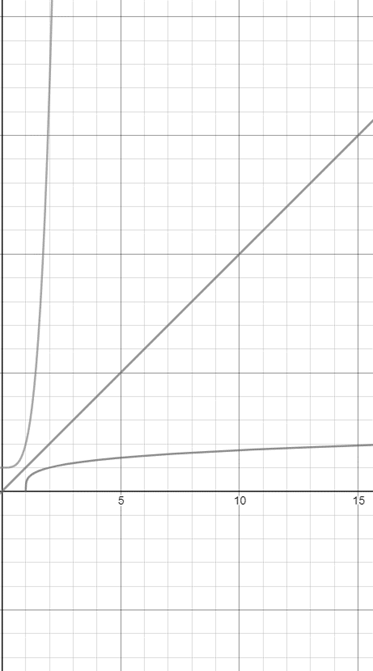# The inverse of the given function and sketch the graph of function and its inverse.### Single Variable Calculus: Concepts...

4th Edition
James Stewart
Publisher: Cengage Learning
ISBN: 9781337687805### Single Variable Calculus: Concepts...

4th Edition
James Stewart
Publisher: Cengage Learning
ISBN: 9781337687805

#### Solutions

Chapter 1.6, Problem 27E
To determine

## To find: The inverse of the given function and sketch the graph of function and its inverse.

Expert Solution

The inverse of the given function is f1(x)=x14 .

### Explanation of Solution

Given information:

The given function is,

f(x)=x4+1

The inverse of the given function is calculated as,

f(x)=x4+1y=x4+1x4=y1x=y14

For the inverse of the function, interchange x and y ,

y=x14f1(x)=x14

Consider the graph of the function and its inverse as follows,Therefore, the inverse of the given function is f1(x)=x14 and the graph of f and f1 are reflection about the line.

### Have a homework question?

Subscribe to bartleby learn! Ask subject matter experts 30 homework questions each month. Plus, you’ll have access to millions of step-by-step textbook answers!